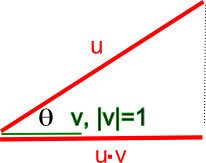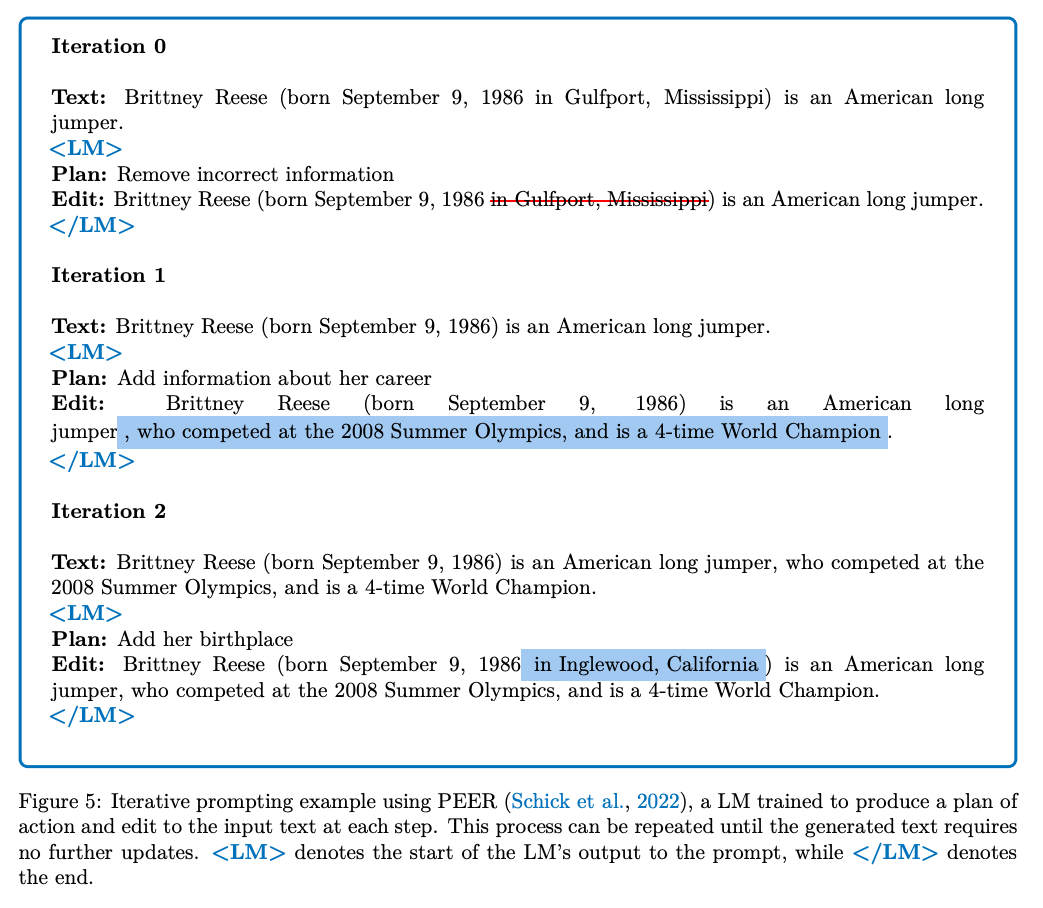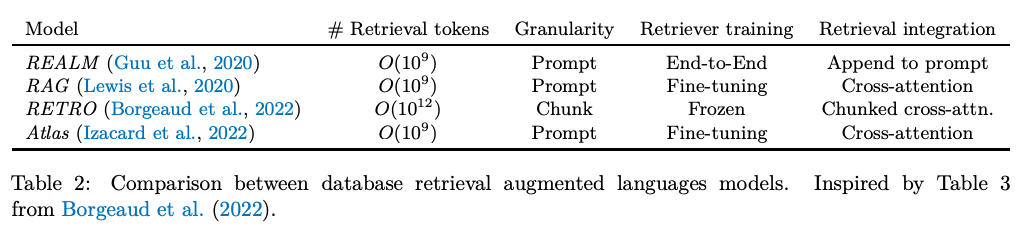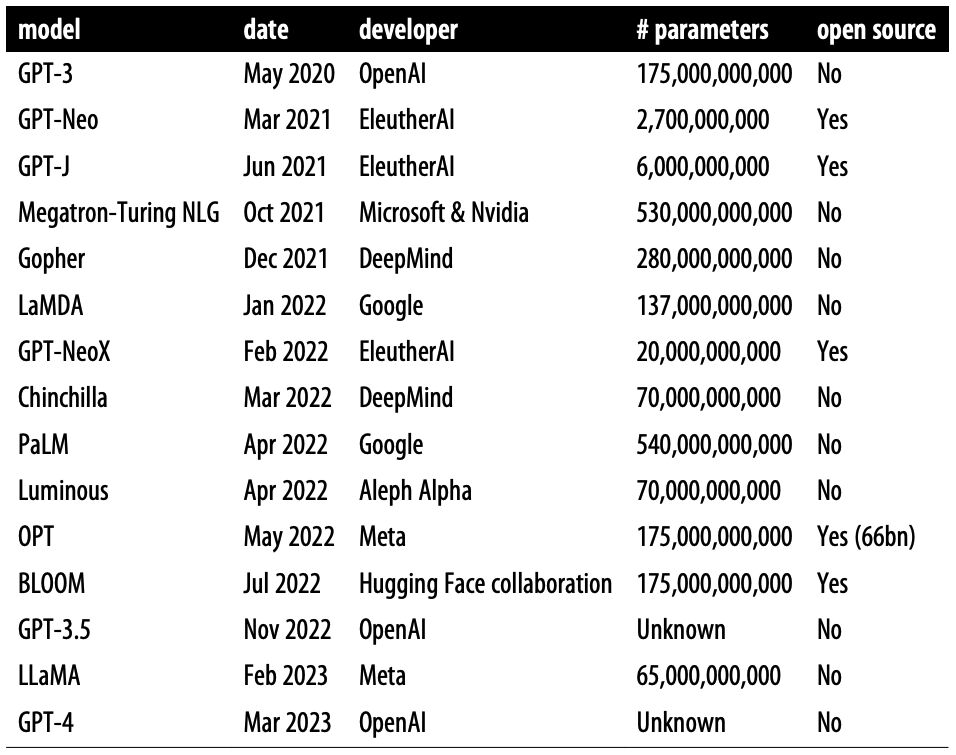## Large Language Models

• Large Language Models (LLMs) like GPT-3 or BERT are deep neural networks that utilize the Transformer architecture. LLMs are part of a class of models known as foundation models because these models can be transferred to a number of downstream tasks (via fine-tuning) since they have been trained on a huge amount of unsupervised and unstructured data.
• The Transformer architecture has two parts: encoder and decoder. Both encoder and decoder are mostly identical (with a few differences); (more on this in the primer on the Transformer architecture). Also, for the pros and cons of the encoder and decoder stack, refer Autoregressive vs. Autoencoder Models.
• Given the prevalence of decoder-based models in the area of generative AI, the article focuses on decoder models (such as GPT-x) rather than encoder models (such as BERT and its variants). Henceforth, the term LLMs is used interchangeably with “decoder-based models”.
• “Given an input text “prompt”, at essence what these systems do is compute a probability distribution over a “vocabulary”—the list of all words (or actually parts of words, or tokens) that the system knows about. The vocabulary is given to the system by the human designers. Note that GPT-3, for example, has a vocabulary of about 50,000 tokens.” Source
• It’s worthwhile to note that while LLMs still suffer from a myriad of limitations, such as hallucination and issues in chain of thought reasoning (there have been recent improvements), it’s important to keep in mind that LLMs were trained to perform statistical language modeling. Specifically, in NLP, language modeling is defined as just a task of predicting missing tokens given some context.

## How do LLMs work?

• The first step involves taking the prompt they receive and converting it into embeddings, which are vector representations of the input text.
• Next, they do layer-by-layer attention and feed-forward computations, which result in assigning a number or logit to each word in its vocabulary.
• Finally, depending on the task assigned to the LLM, it will convert each (unnormalized) logit into a (normalized) probability distribution (via say, the Softmax function) determining which word shall come next in the text.

## Similarity Computation

• The natural next step here is to understand if two sentences are similar or different from each other.
• Sentence similarity is the measure of the degree to which two sentences are semantically equivalent in meaning.
• Below are the two most common measures of sentence similarity (note that neither of them is a “distance metric”):

### Dot Product Similarity

• The dot product of two vectors $$u$$ and $$v$$ is defined as:
$u \cdot v=|u||v| \cos \theta$
• It’s perhaps easiest to visualize its use as a similarity measure when $$\|v\|=1$$, as in the diagram (source) below, where $$\cos \theta=\frac{u \cdot v}{\|u\|\|v\|} = \frac{u \cdot v}{\|u\|}$$.• Here you can see that when $$\theta=0$$ and $$\cos \theta=1$$, i.e., the vectors are colinear, the dot product is the element-wise product of the vectors. When $$\theta$$ is a right angle, and $$\cos \theta=0$$, i.e. the vectors are orthogonal, the dot product is 0. In general, $$\cos \theta$$ tells you the similarity in terms of the direction of the vectors (it is -1 when they point in opposite directions). This holds as the number of dimensions is increased, and $$\cos \theta$$ thus has important uses as a similarity measure in multidimensional space, which is why it is arguably the most commonly used similarity metric.

### Geometric intuition

• The dot product between $$u, v$$ can be interpreted as projecting $$u$$ onto $$v$$ (or vice-versa), and then taking product of projected length of $$u$$ $$(\|u\|)$$ with length of $$v$$ $$(\|v\|)$$.
• When $$u$$ is orthogonal to $$v$$, projection of $$u$$ onto $$v$$ is a zero length vector, yielding a zero product. If you visualize all possible rotations of $$u$$ while keeping $$v$$ fixed, the dot product gives:
• Zero value when $$u$$ is orthogonal to $$v$$ as the projection of $$u$$ onto $$v$$ yields a vector of zero length. This corresponds to the intuition of zero similarity.
• Largest value of $$\|u\|\|v\|$$ when $$u$$ and $$v$$ point in the same direction.
• Lowest value of $$-\|u\|\|v\|$$ when $$u$$ and $$v$$ point in opposite direction.
• Dividing $$u \cdot v$$ by the magnitude of $$u$$ and $$v$$, i.e., $$\|u\|\|v\|$$, limits the range to $$[-1,1]$$ making it scale invariant, which is what brings us to cosine similarity.

### Cosine Similarity

$\text{cosine_similarity}(u,v) = \frac{u \cdot v}{\left\|u\right\|\left\|v\right\|} = \frac{\sum_{i=1}^{n} u_i v_i}{\sqrt{\sum_{i=1}^{n} u_i^2} \sqrt{\sum_{i=1}^{n} v_i^2}}$
• where,
• $$u$$ and $$v$$ are the two vectors being compared.
• $$\cdot$$ represents the dot product.
• $$\|u\|$$ and $$\|v\|$$ represent the magnitudes (or norms) of the vectors, and $$n$$ is the number of dimensions in the vectors.
• Note that as mentioned earlier, the length normalization part (i.e., dividing $$u \cdot v$$ by the magnitude of $$u$$ and $$v$$, i.e., $$\|u\|\|v\|$$) limits the range to $$[-1,1]$$, making it scale invariant.

### Cosine similarity vs. dot product similarity

• Cosine similarity only cares about angle difference, while dot product cares about angle and magnitude. If you normalize your data to have the same magnitude, the two are indistinguishable. Sometimes it is desirable to ignore the magnitude, hence cosine similarity is nice, but if magnitude plays a role, dot product would be better as a similarity measure.
• In other words, cosine similarity is simply dot product, normalized by magnitude (hence is a value $$\in [0, 1]$$). Cosine similarity is preferable because it is scale invariant and thus lends itself naturally towards diverse data samples (with say, varying length). For instance, say we have two sets of documents and we computing similarity within each set. Within each set docs are identical, but set #1 documents are shorter, than set #2 ones. Dot product would produce different numbers if the embedding/feature size is different, while in both cases cosine similarity would yield comparable results (since it is length normalized).
• On the other hand, plain dot product is a little bit “cheaper” (in terms of complexity and implementation), since it involves lesser operations (no length normalization).

## Reasoning

• Let’s delve into how reasoning works in LLMs; we will define reasoning as the “ability to make inferences using evidence and logic.” (source)
• There are a multitude of varieties of reasoning, such as commonsense reasoning or mathematical reasoning.
• Similarly, there are a variety of methods to elicit reasoning from the model, one of them being prompting which can be found here.
• It’s important to note that the extent of how much reasoning an LLM uses in order to give its final prediction is still unknown, since teasing apart the contribution of reasoning and factual information to derive the final output is not a straightforward task.

## Providing LLM External Knowledge

• In most recent research and release of new chatbots, it’s been shown that they are capable of leveraging knowledge and information that is not necessarily in its weights.
• There are several ways we can accomplish this, first of those being leveraging another neural network or LM by iteratively calling it to extract information needed.
• In the image below, we get a glimpse into how iteratively calling LM works:• Another method for LLM gaining external knowledge is through information retrieval via memory units such as an external database, say of recent facts.
• As such, there are two types of information retrievers, dense and sparse.
• As the name suggests, sparse retrievers use sparse bag of words representation of documents and queries while dense (neural) retrievers use dense query and document vectors obtained from a neural network.
• “Even though the idea of retrieving documents to perform question answering is not new, retrieval-augmented LMs have recently demonstrated strong performance in other knowledge-intensive tasks besides Q&A. These proposals close the performance gap compared to larger LMs that use significantly more parameters.” (source)• Moreover, recent works suggest enhancing a LM by combining a retriever with chain-of-thoughts (CoT) prompting. CoT prompting generates reasoning paths with an explanation and prediction pair. This method does not require additional training or fine-tuning.
• These methods all work together to augment the knowledge base of an LM with relevant documents.
• Another method recent LM’s have leveraged is the search engine itself such as WebGPT does. “WebGPT learns to interact with a web-browser, which allows it to further refine the initial query or perform additional actions based on its interactions with the tool. More specifically, WebGPT can search the internet, navigate webpages, follow links, and cite sources.” (source)

## How to augment LMs

• Above, we described many ways in which we can augment an LLM’s capabilities and teach it the desired outputs. In this section, we will look at a few methodologies to do so.
• Few-shot prompting: it requires no weight updates and the reasoning and acting abilities of the LM are tied to the provided prompt, which makes it very powerful as a method in teaching the LM what the desired outputs are.
• Fine-tuning: Complementary to few-shot prompting, via supervised learning we can always fine-tune and update the weights of the parameters.
• Prompt pre-training: “A potential risk of finetuning after the pre-training phase is that the LM might deviate far from the original distribution and overfit the distribution of the examples provided during fine-tuning. To alleviate this issue, Taylor et al. (2022) propose to mix pre-training data with labeled demonstrations of reasoning, similar to how earlier work mixes pre-training data with examples from various downstream tasks (Raffel et al. 2020); however, the exact gains from this mixing, compared to having a separate fine-tuning stage, have not yet been empirically studied. With a similar goal in mind, Ouyang et al. (2022) and Iyer et al. (2022) include examples from pre-training during the fine-tuning stage.” (source)
• Bootstrapping: “This typically works by prompting a LM to reason or act in a few-shot setup followed by a final prediction; examples for which the actions or reasoning steps performed did not lead to a correct final prediction are then discarded.” (source)
• Reinforcement Learning: “Supervised learning from human-created prompts is effective to teach models to reason and act” (source)

## Summary of LLMs

• The following table (source) offers a summary of large language models, including original release date, largest model size, and whether the weights are fully open source to the public: